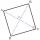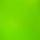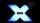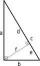I have homework.
The cube has an edge 7 cm long and I must find wall and body diagonal.

Result

d1 =  9.9 cm
d2 =  12.124 cm

#### Solution:Leave us a comment of example and its solution (i.e. if it is still somewhat unclear...):Be the first to comment!#### To solve this example are needed these knowledge from mathematics:

Pythagorean theorem is the base for the right triangle calculator.

## Next similar examples:

1. DogDog is tied to a chain, which is mounted in a corner of the yard. Yard has the shape of a square with a side length of 20 meters. The same long is also dogchain. Are there places in the yard where dog can't reach?
2. Square2Side of the square is a = 6.2 cm, how long is its diagonal?
3. Trapezoid ABCDABDC is a trapezoid in which AB and CD are parallel sides measuring 6 and 9 respectively. Angles ABC and BCD are both right angles. Find the length of segment BD.The ladder has a length 3.5 meters. He is leaning against the wall so that his bottom end is 2 meters away from the wall. Determine the height of the ladder.
5. The cellarMr Novák has a cellar and a cellar window in the chalet has 0.6 meter square window. The window wishes to place an X-shaped grid in a square. He uses iron welded bars. Calculate the lengths of individual bars and what the total length of the bars he has t
6. Four ropesTV transmitter is anchored at a height of 44 meters by four ropes. Each rope is attached at a distance of 55 meters from the heel of the TV transmitter. Calculate how many meters of rope were used in the construction of the transmitter. At each attachment.
7. SquareCalculate area of the square with diagonal 64 cm.
8. TriangleCan be rectangular triangle equilateral?
9. Diagonal of squareCalculate the side of a square when its diagonal is 10 cm.
10. MedianIn the right triangle are sides a=41 dm b=42 dm. Calculate the length of the medians tc to the hypotenuse.
11. Broken treeThe tree was 35 meters high. The tree broke at a height of 10 m above the ground. Top but does not fall off it refuted on the ground. How far from the base of the tree lay its peak?
12. Chord circleThe circle to the (S, r = 8 cm) are different points A, B connected segment /AB/ = 12 cm. AB mark the middle of S'. Calculate |SS'|. Make the sketch.
13. WindbreakA tree at a height of 3 meters broke in the windbreak. Its peak fell 4.5 m from the tree. How tall was the tree?
14. SatinSanusha buys a piece of satin 2.4 m wide. The diagonal length of the fabric is 4m. What is the length of the piece of satin?
15. Broken treeThe tree is broken at 4 meters above the ground and the top of the tree touches the ground at a distance of 5 from the trunk. Calculate the original height of the tree.
16. Trio 2Decide whether trio of numbers is the side of a right triangle: 26,24,10.
17. HostelStudents are accommodated in 22 rooms. Rooms were 4 and 6 bed. How many rooms in which type occupied 106 children there?